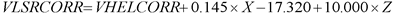Spitzer Documentation & Tools
IRS Instrument Handbook

### 5.1.18         VELOCITY

The module VELOCITY computes Spitzer spacecraft (S/C) velocity components along the line of sight to the observed target. The components it separately computes are: (i) the S/C heliocentric velocity, and (ii) the S/C velocity relative to the Local Standard of Rest (LSR).  These components are written to the header keywords VHELCORR and VLSRCORR, respectively. The values of these keywords can be directly added to Spitzer-measured velocities of astronomical targets, to express them in either heliocentric or LSR reference frames.

The module first computes, at the given Modified Julian Date (read from the keyword MJD_OBS), the S/C velocity components in the S/C coordinate system: VX, VY, and VZ.

Then it computes the quantities X, Y, Z defined as follows:Equation 5.16

where RA_FOV and DEC_FOV are header keywords.

The value of VHELCORR is then the dot product of [X, Y, Z] and [VX, VY, VZ]. The value of VLSRCORR is given by:Equation 5.17